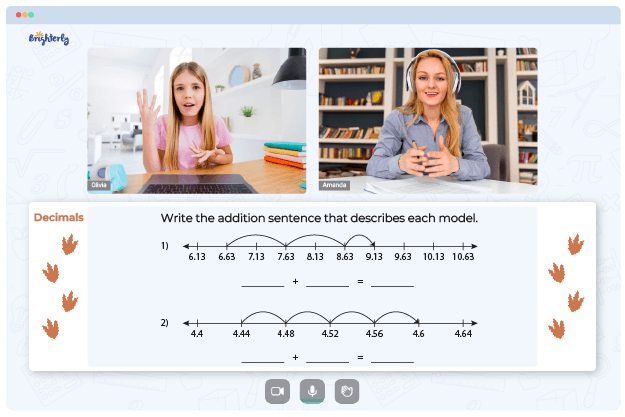# Decimal To Percent Worksheets

Decimals are numbers representing tenths, hundredths, etc., of a unit, while percentages are fractions stated in hundredths-of-a-unit equivalents. Convert decimals to percents worksheets will help your kids understand the fundamentals of mathematics, the knowledge they will use daily for different purposes, for example, figuring out how much something will cost.

Decimals are used for small numbers, whereas percentages are used for larger quantities. These two concepts are utilized frequently to compare numbers or solve problems. There are different kinds of worksheets available for free printing online. However, they are not all the same, and you need to get the best percent to decimal worksheet for your child to get the most out of it.

Math for Kids

Is Your Child Struggling With Math?
1:1 Online Math TutoringIs your child interested in becoming an expert at converting percentages to decimals? Students in middle school are expected to know how to divide a percentage by 100, remove the percent sign, and then write the result as a decimal, which are the typical tasks in printable converting percents to decimals worksheets.### Decimal To Percent Worksheets PDF

Decimal To Percent Worksheet### Decimal To Percent Worksheets PDF

Percent To Decimal Worksheet### Decimal To Percent Worksheets PDF

Convert Decimals To Percents Worksheets### Decimal To Percent Worksheets PDF

Converting Percents To Decimals Worksheets

These listed free printable converting decimals to percents worksheets will equip your child with knowledge on percent-decimal conversion.

### More Percentages Worksheets

Troubles with Decimals?• Does your child struggle to master math decimals?
• Try lessons with an online tutor.

Is your child having trouble grasping of decimals? An online tutor could provide the necessary support.